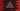# 3 ways in C# to remove elements from an array## C# program to remove elements from an array:

In this post, we will learn different ways to remove an element from an array in C#. C# doesn’t provide any method to remove elements from an array. I will show you 3 different ways to do that in C#.

### Method 1: By using Where:

The Where() clause can be used to filter out elements based on a specific condition. We can use it to remove all elements equal to a given element from an array. Below is the complete program:

``````using System;

namespace Program
{
class Program
{
static void PrintArray<T>(T[] Arr)
{
foreach (T i in Arr)
{
Console.Write(i + " ");
}
Console.WriteLine();
}
static void Main(string[] args)
{
int[] Arr = { 1, 2, 3, 4, 5, 6 };
int[] FinalArr;

int Num = 5;

FinalArr = Arr.Where(N => N != Num).ToArray();

Console.WriteLine("Original array: ");
PrintArray(Arr);

Console.WriteLine("Final array: ");
PrintArray(FinalArr);
}
}
}``````

Here,

• Arr is the original given array. FinalArr is the new array created by removing Num from Arr.
• The Where clause filters all numbers which are not equal to Num and converts the result to an array.
• The last two lines are printing the original and final arrays.

If you run this program, it will print the below output:

``````Original array:
1 2 3 4 5 6
Final array:
1 2 3 4 6``````

### Method 2: By using Array.FindAll:

The Array.FindAll method is another method to delete all unwanted numbers from an array. For example, let’s remove all odd numbers from an array:

``````using System;

namespace Program
{
class Program
{
static void PrintArray<T>(T[] Arr)
{
foreach (T i in Arr)
{
Console.Write(i + " ");
}
Console.WriteLine();
}

static bool IsEven(int n)
{
return n % 2 == 0;
}
static void Main(string[] args)
{
int[] Arr = { 1, 2, 3, 4, 5, 6 };
int[] FinalArr;

int Num = 5;

FinalArr = Array.FindAll(Arr, IsEven);

Console.WriteLine("Original array: ");
PrintArray(Arr);

Console.WriteLine("Final array: ");
PrintArray(FinalArr);
}
}
}``````
• IsEven method returns one boolean value that is true if the passed argument is even, else it is false.
• The Array.FindAll method takes the array as the first parameter and it takes the IsEven method as the second parameter. It will return one array that include all numbers for which IsEven returns True. It will return an array holding all even numbers of Arr.

It will print the below output:

``````Original array:
1 2 3 4 5 6
Final array:
2 4 6``````

### Method 3: By using Array.IndexOf to remove the first instance of a specific element.

The above two examples removes all occurrences of a number or all occurrences that satisfies a given condition. We can also use Array.IndexOf to get the first index of an element in an array and Where can be used to remove the element at that index.

Let me show you with an example:

``````using System;

namespace Program
{
class Program
{
static void PrintArray<T>(T[] Arr)
{
foreach (T i in Arr)
{
Console.Write(i + " ");
}
Console.WriteLine();
}

static void Main(string[] args)
{
int[] Arr = { 1, 2, 3, 4, 5, 6 };
int[] FinalArr;

int Num = 5;

int Index = Array.IndexOf(Arr, Num);
FinalArr = Arr.Where((v, i) => i != Index).ToArray();

Console.WriteLine("Original array: ");
PrintArray(Arr);

Console.WriteLine("Final array: ");
PrintArray(FinalArr);
}
}
}``````

Here, we are finding the index of Num in array Arr. Then we are using Where to remove the element at index Index.